### Home > CAAC > Chapter 8 > Lesson 8.3.1 > Problem8-96

8-96.

Solve the following quadratic equations by factoring and using the Zero Product Property. Be sure to check your solutions. Homework Help ✎

1. $x^2−13x+42=0$

Draw a generic rectangle and diamond.

Factor.

$(x−6)(x−7)=0$

One solution is $x=6$.
Make sure you find the other solution.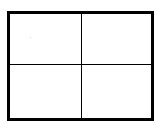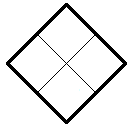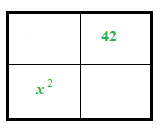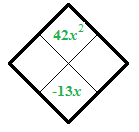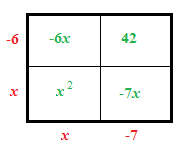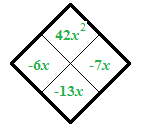1. $0=3x^2+10x−8$

$0=(3x−2)(?\ +\ ?)$

1. $2x^2−10x=0$

There are only $2$ terms, so factor using the GCF.

1. $4x^2+8x−60=0$

Notice that each term is a multiple of $4$.

$x=3$ or $x=-5$